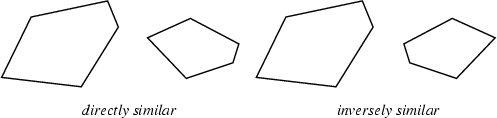## SimilarTwo figures are said to be similar when all corresponding Angles are equal. Two figures are Directly Similar when all corresponding Angles are equal and described in the same rotational sense. This relationship is written. (The symbolis also used to mean is the same order of magnitude as'' and is Asymptotic to.'') Two figures are Inversely Similar when all corresponding Angles are equal and described in the opposite rotational sense.